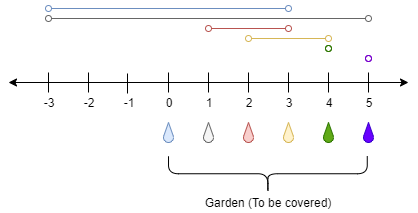##### Welcome to Subscribe On Youtube

Formatted question description: https://leetcode.ca/all/1326.html

# 1326. Minimum Number of Taps to Open to Water a Garden (Hard)

There is a one-dimensional garden on the x-axis. The garden starts at the point 0 and ends at the point n. (i.e The length of the garden is n).

There are n + 1 taps located at points [0, 1, ..., n] in the garden.

Given an integer n and an integer array ranges of length n + 1 where ranges[i] (0-indexed) means the i-th tap can water the area [i - ranges[i], i + ranges[i]] if it was open.

Return the minimum number of taps that should be open to water the whole garden, If the garden cannot be watered return -1.

Example 1:Input: n = 5, ranges = [3,4,1,1,0,0]
Output: 1
Explanation: The tap at point 0 can cover the interval [-3,3]
The tap at point 1 can cover the interval [-3,5]
The tap at point 2 can cover the interval [1,3]
The tap at point 3 can cover the interval [2,4]
The tap at point 4 can cover the interval [4,4]
The tap at point 5 can cover the interval [5,5]
Opening Only the second tap will water the whole garden [0,5]


Example 2:

Input: n = 3, ranges = [0,0,0,0]
Output: -1
Explanation: Even if you activate all the four taps you cannot water the whole garden.


Example 3:

Input: n = 7, ranges = [1,2,1,0,2,1,0,1]
Output: 3


Example 4:

Input: n = 8, ranges = [4,0,0,0,0,0,0,0,4]
Output: 2


Example 5:

Input: n = 8, ranges = [4,0,0,0,4,0,0,0,4]
Output: 1


Constraints:

• 1 <= n <= 10^4
• ranges.length == n + 1
• 0 <= ranges[i] <= 100

Related Topics:
Dynamic Programming, Greedy

## Solution 1.

// OJ: https://leetcode.com/problems/minimum-number-of-taps-to-open-to-water-a-garden/
// Time: O(NlogN)
// Space: O(N)
class Solution {
public:
int minTaps(int n, vector<int>& ranges) {
vector<pair<int, int>> v;
for (int i = 0; i < ranges.size(); ++i)
v.emplace_back(max(0, i - ranges[i]),  min(n, i + ranges[i]));
sort(v.begin(), v.end());
int end = 0, newEnd = 0, ans = 0;
for (int i = 0; i < v.size() && end != n; ++i) {
if (v[i].first > end) return -1;
newEnd = max(newEnd, v[i].second);
if (i + 1 == v.size() || v[i + 1].first > end) {
end = newEnd;
++ans;
}
}
return ans;
}
};

• class Solution {
public int minTaps(int n, int[] ranges) {
int[][] minMaxArray = new int[n + 1];
for (int i = 0; i <= n; i++) {
int range = ranges[i];
int min = Math.max(i - range, 0);
int max = Math.min(i + range - 1, n - 1);
minMaxArray[i] = min;
minMaxArray[i] = max;
}
boolean[] covered = new boolean[n];
int coverCount = 0;
int openCount = 0;
boolean[] used = new boolean[n + 1];
boolean flag = true;
while (flag) {
flag = false;
for (int i = 0; i < n; i++) {
if (covered[i])
continue;
int maxLengthIndex = -1;
int maxLength = 0;
for (int j = 0; j <= n; j++) {
if (used[j])
continue;
int[] array = minMaxArray[j];
int min = array, max = array;
if (min <= i && max >= i) {
int length = 0;
for (int k = min; k <= max; k++) {
if (!covered[k])
length++;
}
if (length > maxLength) {
maxLengthIndex = j;
maxLength = length;
}
}
}
if (maxLengthIndex >= 0) {
used[maxLengthIndex] = true;
int min = minMaxArray[maxLengthIndex], max = minMaxArray[maxLengthIndex];
for (int j = min; j <= max; j++) {
if (!covered[j]) {
covered[j] = true;
coverCount++;
}
}
flag = true;
openCount++;
}
}
}
return coverCount == n ? openCount : -1;
}
}

• // OJ: https://leetcode.com/problems/minimum-number-of-taps-to-open-to-water-a-garden/
// Time: O(NlogN)
// Space: O(N)
class Solution {
public:
int minTaps(int n, vector<int>& ranges) {
vector<pair<int, int>> v;
for (int i = 0; i < ranges.size(); ++i)
v.emplace_back(max(0, i - ranges[i]),  min(n, i + ranges[i]));
sort(v.begin(), v.end());
int end = 0, newEnd = 0, ans = 0;
for (int i = 0; i < v.size() && end != n; ++i) {
if (v[i].first > end) return -1;
newEnd = max(newEnd, v[i].second);
if (i + 1 == v.size() || v[i + 1].first > end) {
end = newEnd;
++ans;
}
}
return ans;
}
};

• class Solution:
def minTaps(self, n: int, ranges: List[int]) -> int:
last =  * (n + 1)
for i, v in enumerate(ranges):
l, r = max(0, i - v), min(n, i + v)
last[l] = max(last[l], r)

ans = mx = pre = 0
for i in range(n):
mx = max(mx, last[i])
if mx <= i:
return -1
if pre == i:
ans += 1
pre = mx
return ans